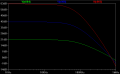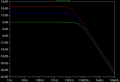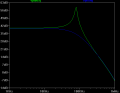# Op amps and gain-bandwidth product

#### tom66

Joined May 9, 2009
2,595
I have wondered about the GBWP (gain-bandwidth product) of an op-amp.

Say I have an LM324 rated at a GBWP = 1.1 MHz. I use it in an amplifier to get 10x amplification, limiting bandwidth to 110 kHz (for now, we'll ignore things like slew rate, PSRR, CMRR, noise etc.) I then stack another LM324 on the output, giving me effectively 100x amplification - but the bandwidth stays the same, because the second amp's bandwidth is only divided by its gain, not the total gain product of the two amplifiers.

Is there a flaw in this logic? Would such a configuration really work?

Just out of curiousity.

#### t06afre

Joined May 11, 2009
5,934
Think of it as signal chain. The weakest link will decide the overall performance.

#### Wendy

Joined Mar 24, 2008
22,775
I know you want to ignore slew rate, but it also directly affects frequency response.

When you look at a chart of the frequency response of an op amp for a gain of 100, you will find the performance is much less than hoped for. An op amp that has unity gain at 1Mhz will have a max gain of 100 at 10Khz (generally it is a gain of 10 every log 10). At 20Khz it will be less, much less. This matches the example you gave nicely.

OK, so you've picked a gain the op amp can handle. With large signals (even sine waves) if it is swinging 10V P-P you will exceed the rate of voltage change that is slew rate for most old op amps. It looks like a freq response issue but isn't. Drop the signal level to something the op amp can handle and it will work fine. Slew rate is important for signal reproduction.

So the highest level of signal response must fall within the slew rate response. Meet this and you can chain op amps indefinitely for more gain.

#### gootee

Joined Apr 24, 2007
447
Good stuff, Bill.

slew rate max of sine (in volts per microsecond) =

[(2 x Pi) x (freq in Hz) x (sine amplitude in volts, zero-to-peak)] / 1,000,000

If the maximum output slew rate of an amplifier is known, the above could be used to calculate the maximum output amplitude (of the maximum expected frequency component) that would keep the amplifier within its maximum output slew rate capability. That would give a limit for the "gain x input amplitude" product. Then the maximum allowed gain could be calculated from the maximum expected input amplitude.

Cheers,

Tom

Last edited:

#### Audioguru

Joined Dec 20, 2007
11,248
The old quad LM324 and its dual, the LM358 were designed for low power so they are extremely slow, very noisy and have crossover distortion. Unlike most other opamps their frequency response and GBP are worse when the supply is less than 30V. The slew rate might also be worse.

#### bitrex

Joined Dec 13, 2009
79
I have wondered about the GBWP (gain-bandwidth product) of an op-amp.

Say I have an LM324 rated at a GBWP = 1.1 MHz. I use it in an amplifier to get 10x amplification, limiting bandwidth to 110 kHz (for now, we'll ignore things like slew rate, PSRR, CMRR, noise etc.) I then stack another LM324 on the output, giving me effectively 100x amplification - but the bandwidth stays the same, because the second amp's bandwidth is only divided by its gain, not the total gain product of the two amplifiers.
I saw this derivation some time back, I think it could be of help.

If you have an amplifier with gain A, then assuming the amplifier has a dominant pole response the gain with respect to frequency is going to be:

$$A(f) = \frac{A}{sqrt{1 + (\frac{\omega}{\omega_o}})^2}$$

Now, if you have a cascade of amplifiers, the total gain is going to be:

$$A*A*A.. = A^n = A_total$$

So the total gain with respect to frequency of the amplifier cascade is going to be:

$$A(f) = (\frac{A_total}{sqrt{1 + (\frac{\omega}{\omega_c}})^2})^n$$

Where n is the number of stages.

The cutoff frequency $$\omega_c$$ of the _cascade_ of amplifiers is going to be:

$$\frac{A_total}{sqrt{1 + (\frac{\omega}{\omega_c}})^n}$$

Setting the two equations equal you can solve for the cutoff frequency of the cascade amplifier:

$$\frac{A_total}{sqrt{1 + (\frac{\omega}{\omega_c}})^2} = (\frac{A}{sqrt{1 + (\frac{\omega}{\omega_o}})^2})^{n}$$

Solving for $$\omega_c$$ you get:

$$\omega_c = \omega_o*\sqrt{2^{\frac{1}{n}}-1}$$

For your two stage amplifier with a bandwidth of 110 kHz each stage, the total bandwidth of both stages combined is 110kHz * 0.643, which is about 70.7 kHz. But because the amplifier has a total gain of 100 times, the gain-bandwidth product of the compound amplifier is therefore 7.07 MHz! (whether you could really get this level of gain at 110Khz with an LM324 is of course, as you say, a question of slew rate and other factors as well.)

Last edited:

#### Audioguru

Joined Dec 20, 2007
11,248
When opamps are combined in series then the GBPs do not multiply. The gain of an opamp at its GBP is 1 so two in series also have a combined GBP that is the same as one opamp.

#### bitrex

Joined Dec 13, 2009
79
When opamps are combined in series then the GBPs do not multiply. The gain of an opamp at its GBP is 1 so two in series also have a combined GBP that is the same as one opamp.
Doesn't follow from the equations above. If the opamp has a GBP of 1 Mhz, then with a gain of 1 it will have a bandwidth of say 1 MHz. A cascade of two of these amplifiers will have a bandwidth of 0.643*1Mhz, so the gain bandwidth product of the combined amplifier will be 1*0.643*1Mhz = 643 kHz. So a cascade of two unity gain buffers actually has a worse GBP than just a single amplifier.

#### Ghar

Joined Mar 8, 2010
655
The GBP doesn't multiply together because at the unity-gain frequency (equal to GBP) the gain is 1; it doesn't matter how many you cascade, if all of them have a gain of 1 your total gain is still 1.

However, the simple relationship of GBP = Gain * BW is only valid for a first-order response. Cascaded op-amps are a higher order system so gain-bandwidth product is meaningless.

With multiple identical op-amp circuits your unity-gain frequency will be the same and your bandwidth (-3dB) will be lower, however you will have higher gain all the way until you get unity-gain frequency.The green plot is one stage, the blue two, and the red three.
The approximate cut-offs (-3dB) are:
w_1 = 75 kHz
w_2 = 44 kHz
w_3 = 36 kHz

Anyway, that derivation is incorrect.
The correct expression is this:
$$w_n = w_o \sqrt{2^{\frac{1}{n}} - 1}$$

With this expression you get:
w_1 = w_o
w_2 = 0.64 w_o
w_3 = 0.51 w_o

This fits the simulation within all my round-off errors...

Of course the gain increases as A^n so the gain increases faster than the bandwidth decreases, it does help the situation.

Last edited:
•bitrex

#### lmartinez

Joined Mar 8, 2009
224
It will be a compromise either the output signal will have a significant amplitude or none at all( to a certain degree).

http://en.wikipedia.org/wiki/Gain-bandwidth_product

#### bitrex

Joined Dec 13, 2009
79
However, the simple relationship of GBP = Gain * BW is only valid for a first-order response. Cascaded op-amps are a higher order system so gain-bandwidth product is meaningless.
But let's say I take the above example of two opamps in cascade, with 100x gain. I then wrap a feedback loop around those two opamps and make a negative feedback amplifier out of the cascade. Surely the compound system is going to have some gain-bandwidth product itself?

#### Ghar

Joined Mar 8, 2010
655
It will be a compromise either the output signal will have a significant amplitude or none at all( to a certain degree).

http://en.wikipedia.org/wiki/Gain-bandwidth_product
That article is awful... that derivation can't say what they claim it says.
If it were indeed a proof of gain-bandwidth product it would apply to all amplifiers when it obviously doesn't.

Gain-bandwidth product isn't a general concept, it only applies in certain cases, the most popular of which is voltage feedback op-amps with a single pole response. It doesn't work when there are more poles and it doesn't work for cascading op-amps because they're each independent. There isn't a tradeoff there.

It doesn't work at all in current feedback op-amps, their gain and bandwidth are independent.

Adding L & C elements does not trade off gain and bandwidth, those break the relationship. Does a bandpass filter follow gain-bandwidth product? What about a PID controller? Those are op-amp circuits with L's and C's...

The origin is pretty simple, this is the general single pole low pass transfer function:
$$A(s) = \frac{A_0}{1 + \frac{s}{w_o}}\\$$

Unity gain frequency:
$$|A(s)| = 1 = \frac{A_0}{sqrt{1 + \frac{w_u^2}{w_o^2}}}\\ w_u = w_0 sqrt{(A_0^2 -1)}$$

(Notice that GBP = A_0 w_o doesn't equal unity frequency unless A0^2 >> 1)

When you add a negative feedback factor of $$\beta$$ you get a closed loop gain of:
$$T(s) = \frac{A(s)}{1 + \beta A(s)}\\ = \frac{\frac{A_0}{1 + \frac{s}{w_o}} }{1 + \beta \frac{A_0}{1 + \frac{s}{w_o}}}\\ = \frac{A_0}{1 + \beta A_0 + \frac{s}{w_o}}\\ =\frac{A_0}{1 + \beta A_0}\cdot\frac{1}{1 + \frac{s}{w_o (1 + \beta A_0)}}$$

Therefore your new pole (bandwidth) is at $$w_0 (1 + \beta A_0)$$ while your new closed loop DC gain is $$\frac{A_0}{1 + \beta A_0}$$

DC gain was reduced by that same factor that bandwidth was increased by so the ratio of DC gain to bandwidth remains constant.

Let's check unity gain frequency again...

$$1 =\frac{A_0}{1 + \beta A_0}\cdot\frac{1}{1 + \frac{w_u}{w_o (1 + \beta A_0)}}\\ w_u = w_0(1 + \beta A_0) sqrt{(\frac{A_0}{1 + \beta A_0})^2 - 1}$$

If your closed loop DC gain squared is much larger than one, then;
$$w_u = w_0 A_0$$

So again GBP relies on an approximation...

If you have two poles (added a capacitor or put two opamps in one loop for example) everything falls apart:

$$A(s) = \frac{A_0}{(1 + \frac{s}{w_1})(1 + \frac{s}{w_2})}$$

Giving you a closed loop gain of:
$$T(s) = \frac{A_0}{\beta A_0 + (1 + \frac{s}{w_1})(1 + \frac{s}{w_2}) }\\ T(s) = \frac{A_0}{\beta A_0 + 1 + \frac{s}{w_1} + \frac{s}{w_2} + \frac{s^2}{w_1 w_2} }\\ T(s) = \frac{A_0}{1 + \beta A_0}\cdot \frac{1}{1 + \frac{s(\frac{1}{w_1} + \frac{1}{w_2}) + \frac{s^2}{w_1 w_2}}{1 + \beta A_0} }$$

You can see the poles will be something complicated now... in fact they could even be a complex conjugate pair. Ever do a root locus of a transfer function? You can see where the poles move when you vary a parameter.

Either way there is no more basis for the simple relationship GBP = A_0 * BW so there's no more reason to talk about it. Unity-gain frequency always exists because it's simply defined as the frequency at which T(s) = 1.

Some simulations from idealized op-amp models;

One pole.Notice the unity gain frequencies aren't equal... this is due to the low gain.

Two poles. Bottom is open loop, top is closed loop with different gains.So the poles became complex conjugate and unity gain frequency is roughly the same as before. However, the bandwidth is completely different.
The open loop has a 3dB of about 15.6 Hz, a unity-gain frequency of 5.2 MHz, and a DC gain of 10^8.
Those do not fit GBP = Gain * BW because of the 2nd pole. It would work however if the 2nd pole came after the unity gain frequency.

In closed loop with highest gain (teal curve) you get a DC gain of 100 with a bandwidth of 325 kHz.

Comparing the two, open loop you had 1.6E9 GBP, while in closed loop you have 3.3E7.
With the lowest gain curve (blue) you have a DC gain of 10 with a bandwidth of 960 kHz. That's 9.6E6 GBP

It doesn't really work.

Since the unity gain frequencies are still the same and knowing that you have the 40 dB/dec drop approaching it you could calculate the bandwidth but it's not related to the DC gain anymore.

Here's a simulation with two different LT1001 circuits.
Blue is simply a cascade of 2 non-inverting configurations each with a gain of 10, while green is 2 op-amps inside a non-inverting configuration with overall feedback for a gain of 10.It actually gave a resonant peak in the response for the op-amps with overall feedback.
The cascaded op-amp amplifiers can't do that because they're independent so there's no overall relationship. Notice both have the same unity gain frequency still.

I am tired at this point so if I'm missing something someone please point it out...

#### tom66

Joined May 9, 2009
2,595
I'm just wondering why they wouldn't multiply.

For now, let's assume the op-amps are completely independent and ideal in every way except their GBWP.

The first amp takes a 100mVp-p signal @ 100 kHz and amplifies it with a gain of 10. This gives us a 1Vp-p signal @ 100 kHz. The second amp does the same, giving us 10Vp-p @ 100 kHz. This is equivalent to feeding a 1Vp-p signal @ 100 kHz into the same amplifier from an independent source, and so it shouldn't make any difference.

The two parts are independent, so the overall GBWP should be 100 kHz, but the gain should be 100, giving an effective GBWP of 10 MHz.

#### Ghar

Joined Mar 8, 2010
655
Gainbandwidth product is a very specific thing where it implies that the gain times the bandwidth is a constant. If it isn't constant there is no use talking about the product of gain and bandwidth (at least in my opinion).